Skip to main content Skip to Search Box

Definition: subtraction from The Penguin Dictionary of Science

The mathematical operation in which the difference of two numbers, vectors, matrices, polynomials, etc., is found. It is best thought of as the inverse of ➤addition.

Summary Article: Lesson 1-3: Subtraction
from Homework Helpers: Basic Math and Pre-Algebra

The symbol for subtraction is -. The number to be subtracted from is called the minuend, and the number to be subtracted is called the subtrahend. The result of the subtraction is called the difference. The symbol for what the difference is equal to is the equal sign, =.

Subtraction is the reverse of addition. If 5 + 3 = 8 then 8 - 3 = 5 and 8 - 5 = 3. You can always check your answers by adding the difference and the subtrahend together; their sum should be the minuend.

Even though subtraction is the reverse of addition, there are some differences. With subtraction, the order does matter; subtraction is not commutative. We also cannot group things arbitrarily; subtraction is not associative. We will talk more about the order in which we calculate in Lesson 1-8.

Sometimes subtraction is written horizontally: 8 - 5 = 3. Other times subtraction is written vertically: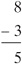The vertical representation is particularly useful when you are subtracting two numbers with two or more digits. When you subtract one two-digit number from another, you can write the two numbers in a column format with the ones, tens, hundreds, and so on aligned. Subtract each column of digits; if the top digit is smaller than the bottom digit, you need to borrow from the column to the left.

Example 1

Find the difference: 68 - 35

Solution: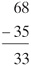You should make a point of checking your work: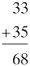Example 2

Find the difference: 54 - 29

Solution: When subtracting the numbers in the ones' place, we have 4 - 9. Because 4 is less than 9, we will need to borrow a 10 from the tens' place and the number in the tens' place will be reduced from 5 to 4: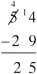Again, check your work: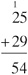Example 3

Find the difference: 156 - 68

Solution: When subtracting the numbers in the ones' place, we have 6 - 8. Because 6 is less than 8, we will need to borrow a 10 from the tens' place and the number in the tens' place will be reduced from 5 to 4. Looking ahead to the subtraction in the tens' place, we will have 4 - 6. Because 4 is less than 6, we will also need to borrow a 100 from the hundreds’ place: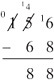Check your work: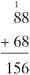Lesson 1-3 Review

Find the following differences.

1. 9 - 2

2. 72 - 31

3. 63 - 39

4. 322 - 78Copyright 2011 © by Denise Szecsei

### Related Articles

##### Full text Article subtraction
The Penguin Dictionary of Mathematics

The inverse operation to addition; the process, for two given numbers, of finding a third which, added to one of the numbers, gives the other,...

##### Full text Article subtract
Word Origins

[16 century] To subtract something is etymologically to ‘pull it away’. The word comes from subtractus , the past participle of Latin ...

##### Full text Article subtraction
Dictionary of Business

/səb‘trækən/ noun An act of taking one number away from another ...

See more from Credo